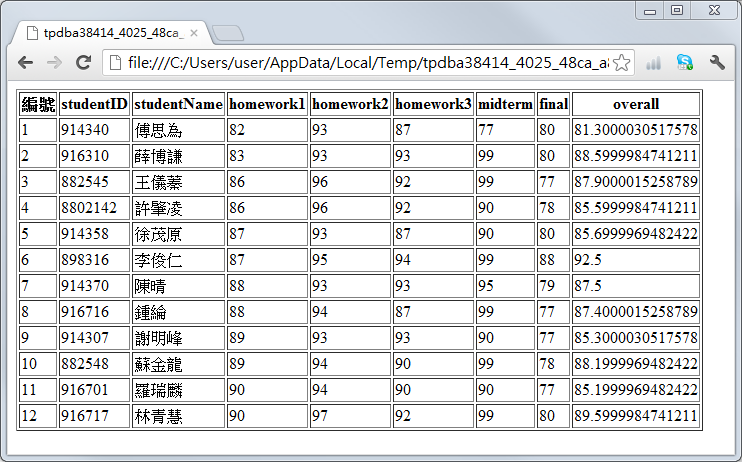## 13-5 雿輻鍂MATLAB撠滩??坔澈?脰?靽格㺿

INSERT INTO score (studentID, studentName, final) VALUES (''00'', ''大力水手'', 100)

Example 1: 13-與資料庫的整合/insertData01.mcopyfile('score01.mdb', 'score02.mdb'); % 將 score01.mdb 拷貝到 score02.mdb dsn = 'dsnScore02'; % 設定資料來源名稱（指到 score02.mdb） logintimeout(5); % 設定嘗試連結資料庫的時間 conn = database(dsn, '', ''); % 連結資料庫 % 設定新增資料所用的 SQL 命令 sql = 'INSERT INTO score (studentID, studentName, final) VALUES (''00'', ''大力水手'', 100)'; cursor = exec(conn, sql); % 執行 SQL 命令 % 設定查詢資料用的 SQL 命令 sql = 'select * from score'; cursor = exec(conn, sql); cursor = fetch(cursor); newScore = cursor.data % 顯示更新後 final 欄位的資料 close(cursor); % 結束 cursor 物件 close(conn); % 結束資料庫連結newScore = '914340' '傅思為'  [ 93] [ 87] [ 77] [ 80]  '916310' '薛博謙'  [ 93] [ 93] [ 99] [ 80]  '882545' '王儀蓁'  [ 96] [ 92] [ 99] [ 77]  '8802142' '許肇凌'  [ 96] [ 92] [ 90] [ 78]  '914358' '徐茂原'  [ 93] [ 87] [ 90] [ 80]  '898316' '李俊仁'  [ 95] [ 94] [ 99] [ 88]  '914370' '陳晴'  [ 93] [ 93] [ 95] [ 79]  '916716' '鍾綸'  [ 94] [ 87] [ 99] [ 77]  '914307' '謝明峰'  [ 93] [ 93] [ 90] [ 77]  '882548' '蘇金龍'  [ 94] [ 90] [ 99] [ 78]  '916701' '羅瑞麟'  [ 94] [ 90] [ 90] [ 77]  '916717' '林青慧'  [ 97] [ 92] [ 99] [ 80]  '00' '大力水手' [ 0] [NaN] [NaN] [NaN]  

UPDATE score SET final=100 WHERE final>=80

Example 2: 13-與資料庫的整合/updateData01.mcopyfile('score01.mdb', 'score02.mdb'); % 將 score01.mdb 拷貝到 score02.mdb dsn = 'dsnScore02'; % 設定資料來源名稱（指到 score02.mdb） logintimeout(5); % 設定嘗試連結資料庫的時間 conn = database(dsn, '', ''); % 連結資料庫 % 設定更新資料所用的 SQL 命令 sql = 'UPDATE score SET final=100 WHERE final>=80'; cursor = exec(conn, sql); % 執行 SQL 命令 % 設定進行資料查詢的 SQL 命令 sql = 'select studentName, final from score'; % 設定 SQL 命令 cursor = exec(conn, sql); cursor = fetch(cursor); newScore = cursor.data % 顯示更新後 final 欄位的資料 close(cursor); % 結束 cursor 物件 close(conn); % 結束資料庫連結newScore = '傅思為'  '薛博謙'  '王儀蓁' [ 77] '許肇凌' [ 78] '徐茂原'  '李俊仁'  '陳晴' [ 79] '鍾綸' [ 77] '謝明峰' [ 77] '蘇金龍' [ 78] '羅瑞麟' [ 77] '林青慧' 

DELETE FROM score WHERE final<80

Example 3: 13-與資料庫的整合/deleteData01.mcopyfile('score01.mdb', 'score02.mdb'); % 將 score01.mdb 拷貝到 score02.mdb dsn = 'dsnScore02'; % 設定資料來源名稱（指到 score02.mdb） logintimeout(5); % 設定嘗試連結資料庫的時間 conn = database(dsn, '', ''); % 連結資料庫 % 設定刪除資料所用的 SQL 命令 sql = 'DELETE FROM score WHERE final<80'; cursor = exec(conn, sql); % 設定進行資料查詢的 SQL 命令 sql = 'select studentName, final from score'; cursor = exec(conn, sql); cursor = fetch(cursor); newScore = cursor.data % 顯示更新後 final 欄位的資料 close(cursor); % 結束 cursor 物件 close(conn); % 結束資料庫連結newScore = '傅思為'  '薛博謙'  '徐茂原'  '李俊仁'  '林青慧' 

Example 4: 13-與資料庫的整合/createTable01.mcopyfile('score01.mdb', 'score02.mdb'); % 將 score01.mdb 拷貝到 score02.mdb dsn = 'dsnScore02'; % 設定資料來源名稱（指到 score02.mdb） logintimeout(5); % 設定嘗試連結資料庫的時間 conn = database(dsn, '', ''); % 連結資料庫 % 產生新資料表 friend sql = 'CREATE TABLE friend (fullName char(6), birthday date)'; exec(conn, sql); % 插入第一筆資料 sql = 'INSERT INTO friend (fullName, birthday) VALUES (''王瓊雯'', ''1983/11/03'')'; exec(conn, sql); % 插入第二筆資料 sql = 'INSERT INTO friend (fullName, birthday) VALUES (''葉佳慧'', ''1982/09/22'')'; exec(conn, sql); % 列出所有資料 cursor = exec(conn, 'select * from friend'); cursor = fetch(cursor); friend = cursor.data % 顯示更新後 friend 資料表的資料 close(cursor); % 結束 cursor 物件 close(conn); % 結束資料庫連結friend = '王瓊雯' '1983-11-03 00:00:00.0' '葉佳慧' '1982-09-22 00:00:00.0'

1. 讀入 score02.mdb 的 score 資料表
2. 計算每一個學生的期末總成績，等於作業平均乘以 30%，加上期中考成績（midterm 欄位）乘以 30%，再加上期末考（final 欄位）成績乘以 40%。
3. 將每一個學生的期末成績送到資料庫的 overall 欄位。
4. 將最後成績計算結果寫成一個網頁，以便公布給學生看。

Example 5: 13-與資料庫的整合/computeScore01.mcopyfile('score01.mdb', 'score02.mdb'); % 將 score01.mdb 拷貝到 score02.mdb dsn = 'dsnScore02'; % 設定資料來源名稱（指到 score02.mdb） logintimeout(5); % 設定嘗試連結資料庫的時間 conn = database(dsn, '', ''); % 連結資料庫 % 抓出所有的資料 cursor = exec(conn, 'select * from score'); % 執行 SQL 命令，並傳回 cursor 物件 cursor = fetch(cursor); % 經由 cursor 物件，抓取全部資料 score = cursor.data; % 將資料傳至 MATLAB 變數 score temp = columnnames(cursor); % 資料庫欄位名稱 eval(['fieldNames = {', temp, '}'';']); % 將欄位名稱指定到 fieldNames 變數 score = cell2struct(score, fieldNames, 2); % 將異質陣列 score 轉成結構陣列 % 對每一筆資料進行運算，並將結果存回資料庫 for i=1:length(score) homework=(score(i).homework1+score(i).homework2+score(i).homework3)/3; overallScore=homework*0.3+score(i).midterm*0.3+score(i).final*0.4; % 將資料寫入資料庫 sql = ['UPDATE score SET overall=', num2str(overallScore), ' where studentID=''', score(i).studentID, '''']; exec(conn, sql); end % 列出 score 資料表 cursor = exec(conn, 'select * from score'); cursor = fetch(cursor); newScore = cursor.data; % 更新後的資料 temp = columnnames(cursor); % 資料庫欄位名稱 eval(['fieldNames = {', temp, '}'';']); % 將欄位名稱指定到 fieldNames 變數 newScore = cell2struct(newScore, fieldNames, 2);% 將異質陣列 newScore 轉成結構陣列 close(cursor); % 結束 cursor 物件 close(conn); % 結束資料庫連結 struct2html(newScore); % 顯示結構陣列 newScore 於瀏覽器MATLAB程式設計：進階篇